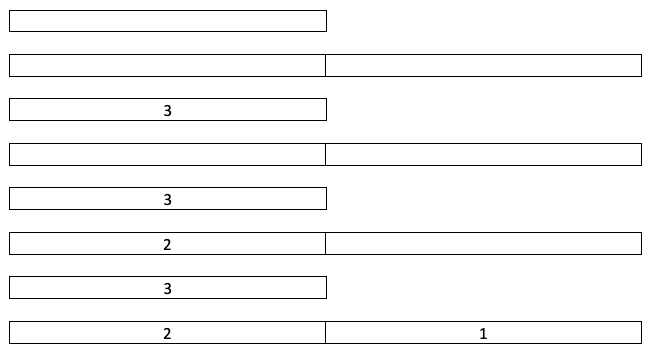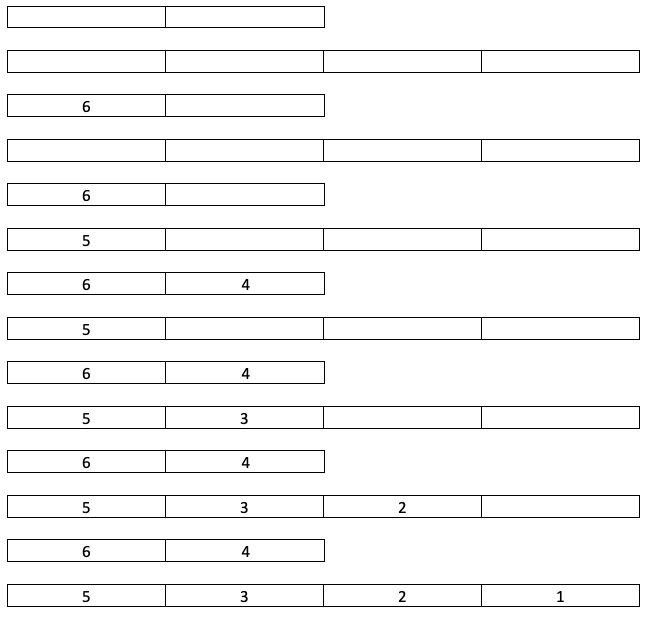×

Search anything:

# Divide numbers from 1 to n into two groups with minimum sum difference from O(2^N) to O(N)

#### Algorithms Greedy AlgorithmsFor numbers from 1 to given n, we have to find the division of the elements into two groups having minimum absolute sum difference. We will explore two techniques:

• Brute force which will take O(2^N) time complexity
• Greedy algorithm which will take O(N) time complexity

Hence, we have reduced an exponential time complexity O(2^N) to a linear time complexity O(N).

### Naive Approach `O(2^N)`

A naive approach would be to generate all subsets of the array of length N and calculating the difference for each of the two subsets in them. Since generating all subsets takes exponential time, this approach is very inefficient.

The steps involved are:

1. Generate all subsets of the array and their corresponding complements.
2. Calculate the sum of all the elements over the two subsets.
3. Compute the absolute sum difference between the two subsets.
4. Update the minimum value of the absolute difference.

Pseudocode:

``````Input: Set[], set_size
1. Get the size of power set
powet_set_size = pow(2, set_size)
min_sum = INT_MAX
2  Loop for counter from 0 to pow_set_size
(a) Loop for i = 0 to set_size
(i) Initialize temp_sum to 0
(ii) If ith bit in counter is set
Print ith element from set for this subset
Update temp_sum by summing with ith element and taking difference with complement sum
(iii) Set min_sum to min(min_sum, temp_sum)
(b) Print seperator for subsets i.e., newline
``````

### Greedy Algorithm `O(N)`

We can divide numbers from 1 to n into two groups such that their absolute sum difference is always 1 or 0. So the absolute difference is atmost 1.

We maintain two counters a and b, both of them initialized to 0 and one temporary variable initialized to half of the sum of the elements.

1. Run a loop from n to 1.
2. If the element does not exceed sum, insert it into Group 1, increment a by the element and decrement sum by the element.
3. Else, insert the element into Group 2.

### Implementation

``````#include <bits/stdc++.h>

using namespace std;

int main()
{
//Obtaining bound of n
int n;
cin >> n;

//Setting half sum value
int sum = (n*(n+1)/2)/2;

//Initializing counter variable
int a = 0, b = 0;

//Maintaining two groups
vector<int> group1, group2;

//Running loop from n to 1
for(int i=n;i>=1;i--){
if(sum-i>=0){
group1.push_back(i);
sum -= i;
a += i;
}else{
group2.push_back(i);
b += i;
}
}

//Printing minimum sum difference
cout << "Minimum difference: " << abs(a-b) << endl;

//Printing the elements of the two groups
cout << "Size of Group 1: " << group1.size() << endl;
for(int i=0;i<group1.size();i++){
cout << group1[i] << " ";
}
cout << endl;
cout << "Size of Group 2: " << group2.size() << endl;
for(int i=0;i<group2.size();i++){
cout << group2[i] << " ";
}

return 0;
}
``````

### Examples

``````//Console input
3
//Console output
Minimum difference: 0
Size of Group 1: 1
3
Size of Group 2: 2
2 1
``````

Here is the state of the two groups for all iterations:• Here sum is initialized to 3. Since sum - 3 is 0, 3 is inserted into Group 1. For each subsequent element, sum - i is less than 0, so it is inserted into Group 2.
``````//Console input
6
//Console output
Minimum difference: 1
Size of Group 1: 2
6 4
Size of Group 2: 4
5 3 2 1
``````

Here is the state of the two groups for all iterations:• Here sum is initialized to 10. Since sum - 6 is 4, 6 is inserted into Group 1. Since sum - 5 is less than 0, it is inserted into Group 2. Since sum - 4 is 0, it is inserted into Group 1. For each subsequent element, sum - i is less than 0, so it is inserted into Group 2.

### Complexity

The time complexity of this algorithm is `O(N)`, as we loop from N to 1.